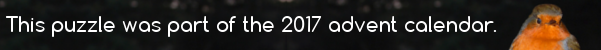mscroggs.co.uk
mscroggs.co.uksubscribe

# Puzzles

## 6 December$$p(x)$$ is a quadratic with real coefficients. For all real numbers $$x$$,
$$x^2+4x+14\leq p(x)\leq 2x^2+8x+18$$
$$p(2)=34$$. What is $$p(6)$$?

Source: Luciano Rila (@DrTrapezio)
$$p(x)$$ is a quadratic polynomial with real coefficients. For all real numbers $$x$$,
$$x^2-2x+2\leq p(x)\leq 2x^2-4x+3$$
$$p(11)=181$$. Find $$p(16)$$.

## Parabola

On a graph of $$y=x^2$$, two lines are drawn at $$x=a$$ and $$x=-b$$ (for $$a,b>0$$. The points where these lines intersect the parabola are connected.
What is the y-coordinate of the point where this line intersects the y-axis?

## Archive

Show me a random puzzle
▼ show ▼